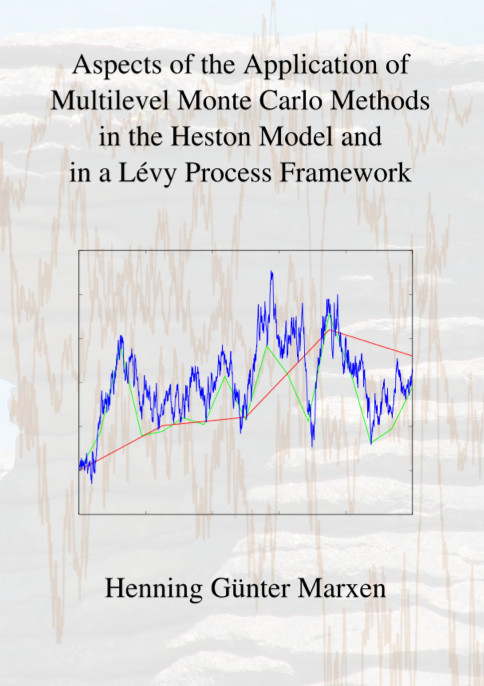Datenbestand vom 20. August 2019aktualisiert am 20. August 2019

# ISBN 9783843906364Euro 72,00 inkl. 7% MwSt

978-3-8439-0636-4, Reihe Mathematik

Henning Marxen
Aspects of the Application of Multilevel Monte Carlo Methods in the Heston Model and in a Lévy Process Framework

159 Seiten, Dissertation Technische Universität Kaiserslautern (2012), Hardcover, B5

## Zusammenfassung / Abstract

This thesis deals with simulation problems concerning the Multilevel Monte Carlo method. These problems are addressed both in a practical and a theoretical setting.

The general question addressed can be seen as the valuation of an expected value of some function on the solution of a stochastic differential equation. This problem is not only arising in the financial mathematical field but has applications in different areas such as physics or electrical engineering.

The thesis is structured as follows. The first chapter gives an overview over well-known results in the field of the Monte Carlo method and introduces important aspects and concepts used later on.

The second chapter deals with general questions concerning the Multilevel Monte Carlo method.

Besides presenting the Multilevel Monte Carlo method, the relation to the statistical Romberg method is analysed. Furthermore, an start level optimization is introduced which turns out to be essential for the practicability of the Multilevel method.

For the pricing of barrier options in the Heston setting, insufficient theoretical results are known to determine an efficient algorithm.

Therefore, in Chapter 3, a new practical approach is used to analyze the simulation methods for double barrier options in the Heston model.

Chapter 4 follows a more theoretical approach. In the setting of Lévy driven stochastic differential equations which allow jumps, several approximate schemes used with multilevel methods are presented.

For known methods, partially new intuitive proofs are provided which help to understand the procedure.

New strong convergence results are shown.